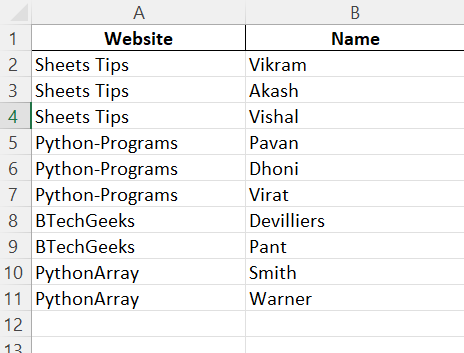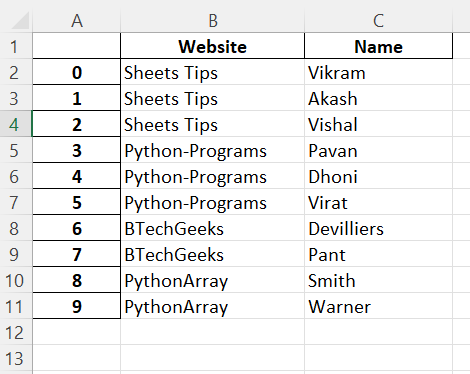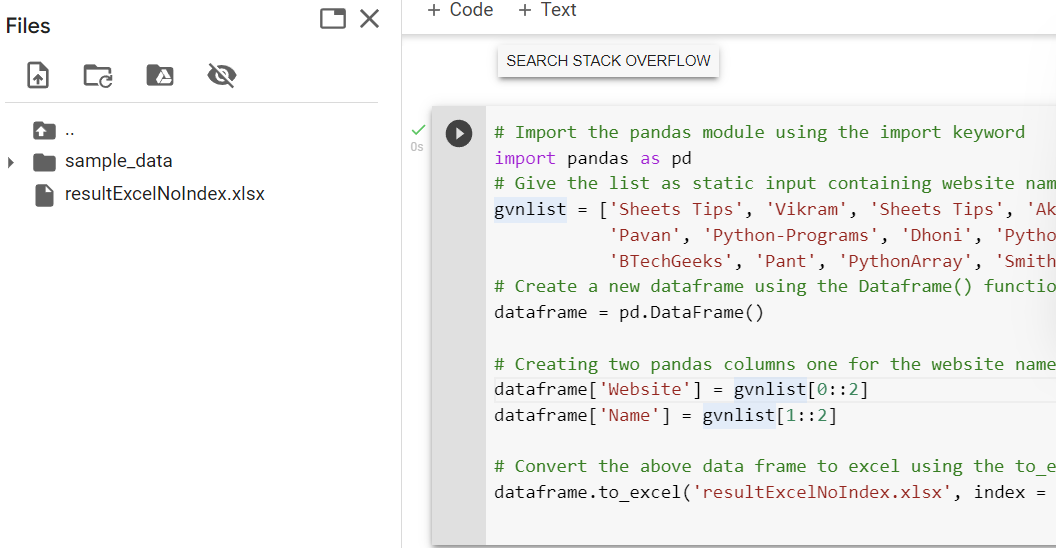# Python Split given List and Insert in Excel File – List to Excel Python Pandas

List To Excel using Python Pandas: If you are searching for a tutorial on how to split a given list in Python and insert it into Excel using Python Pandas, then this page is for you. Using Python Pandas, it is very easy to split the given text into columns and insert the columns into the Excel file. Pandas use the function called df.to_excel() to insert the data into Excel using Python. For example, let us assume that you have a dataset of names and their respective addresses. Using Pandas, you can simply create a column for names and another column with their respective addresses. To learn how to execute this in Python, read this page. This page contains a detailed explanation of how to insert a list in Excel using Python. Scroll down to find out more.

## Python Split Given List and Insert in Excel File Using Pandas

Given a Python list containing website names with their corresponding authors, the task is to split and convert the given list into an excel file in Python.

Prerequisites:

## Split given List and Insert in Excel File in Python

Below are the ways to split the given list and insert in Excel File in Python:

### Method #1: Using Pandas With No Index (Static Input)

Pandas, a popular data analysis library, can be used. We can easily alter the columns in pandas and simply put the filtered components into an excel file using the df.to_excel() function.

to_excel() function:

```To export the DataFrame to an excel file, use the to_excel() method. The target file name must
be specified when writing a single object to an excel file. If we wish to write to many sheets,
we must first construct an ExcelWriter object with the target filename and then indicate the
sheet in the file we want to write to. The unique sheet name can also be used to write numerous
sheets. All modifications made to the data written to the file must be saved.```

Approach:

• Import the pandas module using the import keyword.
• Give the list as static input containing website names with their corresponding authors.
• Create a new dataframe using the Dataframe() function of the pandas module and store it in a variable.
• Creating two pandas columns one for the website name and the second for an author to split the given list.
• Convert the above data frame to excel using the to_excel() function by setting the index as False.
• The Exit of the Program.

Below is the Implementation:

```# Import the pandas module using the import keyword
import pandas as pd
# Give the list as static input containing website names with their corresponding authors.
gvnlist = ['Sheets Tips', 'Vikram', 'Sheets Tips', 'Akash', 'Sheets Tips', 'Vishal', 'Python-Programs',
'Pavan', 'Python-Programs', 'Dhoni', 'Python-Programs', 'Virat', 'BTechGeeks', 'Devilliers',
'BTechGeeks', 'Pant', 'PythonArray', 'Smith', 'PythonArray', 'Warner']
# Create a new dataframe using the Dataframe() function of the pandas module and store it in a variable.
dataframe = pd.DataFrame()

# Creating two pandas columns one for the website name and the second for an author to split the given list.
dataframe['Website'] = gvnlist[0::2]
dataframe['Name'] = gvnlist[1::2]

# Convert the above data frame to excel using the to_excel() function by setting the index as False.
dataframe.to_excel('resultExcelNoIndex.xlsx', index = False)
```

Output:### Method #2: Using Pandas With Index (Static Input)

Approach:

• Import the pandas module using the import keyword.
• Give the list as static input containing website names with their corresponding authors.
• Create a new dataframe using the Dataframe() function of the pandas module and store it in a variable.
• Creating two pandas columns one for the website name and the second for an author to split the given list.
• Convert the above data frame to excel using the to_excel() function by setting the index as True.
• The Exit of the Program.

Below is the Implementation:

```# Import the pandas module using the import keyword
import pandas as pd
# Give the list as static input containing website names with their corresponding authors.
gvnlist = ['Sheets Tips', 'Vikram', 'Sheets Tips', 'Akash', 'Sheets Tips', 'Vishal', 'Python-Programs',
'Pavan', 'Python-Programs', 'Dhoni', 'Python-Programs', 'Virat', 'BTechGeeks', 'Devilliers',
'BTechGeeks', 'Pant', 'PythonArray', 'Smith', 'PythonArray', 'Warner']
# Create a new dataframe using the Dataframe() function of the pandas module and store it in a variable.
dataframe = pd.DataFrame()

# Creating two pandas columns one for the website name and the second for an author to split the given list.
dataframe['Website'] = gvnlist[0::2]
dataframe['Name'] = gvnlist[1::2]

# Convert the above data frame to excel using the to_excel() function by setting the index as False.
dataframe.to_excel('resultExcelWithIndex.xlsx', index = True)
```

Output:### Method #3: Using Pandas Without Index (User Input)

Approach:

• Import the pandas module using the import keyword
• Give the list as user input using list(),input() and split() functions containing website names with their corresponding authors.
• Create a new dataframe using the Dataframe() function of the pandas module and store it in a variable.
• Creating two pandas columns one for the website name and the second for an author to split the given list.
• Convert the above data frame to excel using the to_excel() function by setting the index as False.
• The Exit of the Program.

Below is the Implementation:

```# Import the pandas module using the import keyword
import pandas as pd
# Give the list as user input using list(),input() and split() functions containing website names with their corresponding authors.
gvnlist = list(input('Enter the data : ').split())
# Create a new dataframe using the Dataframe() function of the pandas module and store it in a variable.
dataframe = pd.DataFrame()

# Creating two pandas columns one for the website name and the second for an author to split the given list.
dataframe['Website'] = gvnlist[0::2]
dataframe['Name'] = gvnlist[1::2]

# Convert the above data frame to excel using the to_excel() function by setting the index as False.
dataframe.to_excel('resultExcelWithIndex.xlsx', index = False)
```

Input:

```SheetsTips Vikram SheetsTips Akash SheetsTips Vishal Python-Programs Pavan Python-Programs
Dhoni Python-Programs Virat BTechGeeks Devilliers BTechGeeks Pant PythonArray Smith
PythonArray Warner```

Output:### Method #4: Using Pandas With Index (User Input)

Approach:

• Import the pandas module using the import keyword
• Give the list as user input using list(),input() and split() functions containing website names with their corresponding authors.
• Create a new dataframe using the Dataframe() function of the pandas module and store it in a variable.
• Creating two pandas columns one for the website name and the second for an author to split the given list.
• Convert the above data frame to excel using the to_excel() function by setting the index as True.

Below is the Implementation:

```# Import the pandas module using the import keyword
import pandas as pd
# Give the list as user input using list(),input() and split() functions containing website names with their corresponding authors.
gvnlist = list(input('Enter the data : ').split())
# Create a new dataframe using the Dataframe() function of the pandas module and store it in a variable.
dataframe = pd.DataFrame()

# Creating two pandas columns one for the website name and the second for an author to split the given list.
dataframe['Website'] = gvnlist[0::2]
dataframe['Name'] = gvnlist[1::2]

# Convert the above data frame to excel using the to_excel() function by setting the index as true.
dataframe.to_excel('resultExcelWithIndex.xlsx', index = True)
```

Input:

```SheetsTips Vikram SheetsTips Akash SheetsTips Vishal Python-Programs Pavan Python-Programs
Dhoni Python-Programs Virat BTechGeeks Devilliers BTechGeeks Pant PythonArray Smith
PythonArray Warner```

Output: